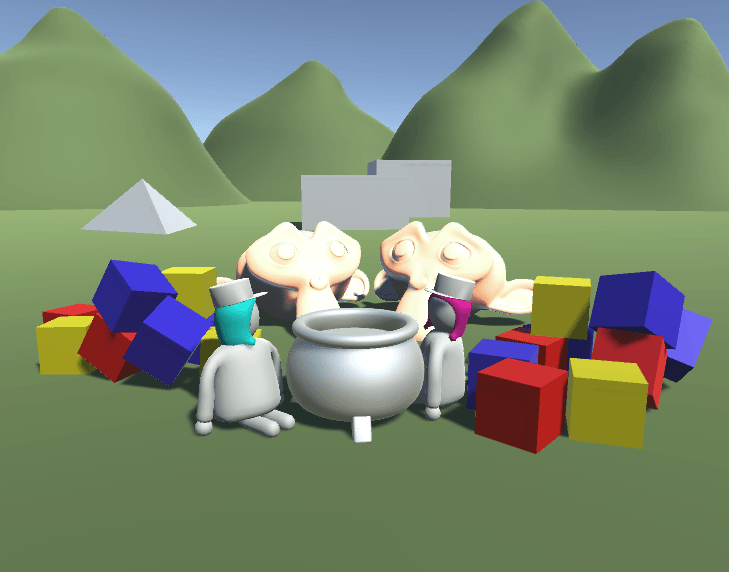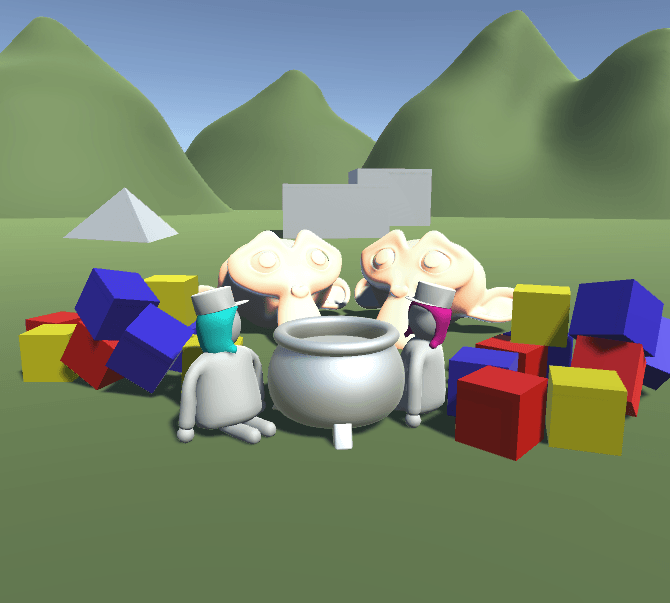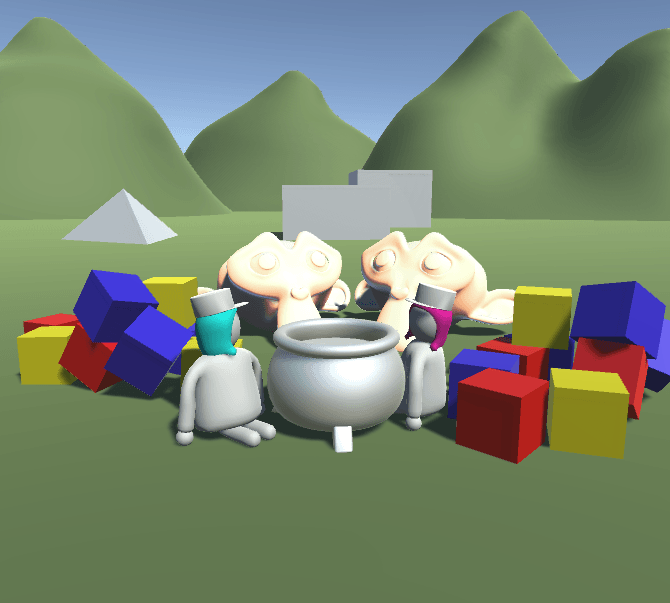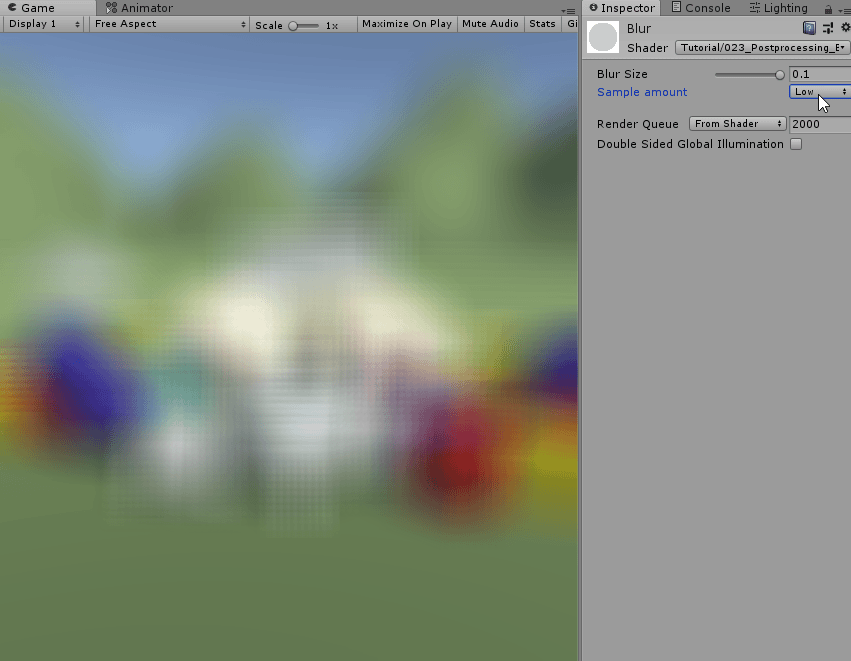## Summary

A effect that’s useful for example to show exhaustion or to make transitions is a blur. To blur the screen we take the average of the surrounding pixels. You can use the effect in many places, but the easiest and most straightforward is probably as a postprocessing effect, so it’s best for you to know how to write postprocessing effects before doing this tutorial.## Boxblur

The easiest form of a blur is a box blur, it just takes the average of a square area and displays it. To access the many different points on the source texture we just iterate over them with a for loop. After reading the color at the different positions we add it to a color variable. And then after adding all of the texture colors we divide by the amount of samples we added to get the average.

We can use the shader with the postprocessing script we made for the previous tutorial.

``````//the fragment shader
fixed4 frag(v2f i) : SV_TARGET{
//init color variable
float4 col = 0;
for(float index=0;index<10;index++){
//add color at position to color
col += tex2D(_MainTex, i.uv);
}
//divide the sum of values by the amount of samples
col = col / 10;
return col;
}
``````

### 1D Blur

Because we’re reading the texture 10 times at the same point, our shader doesn’t change anything yet, so the next step is to actually read from different positions on the screen. For this we add a new property and global variable called blur size. This way we can change how much the shader will blur the image. The variable will change the size of the rectangle we take the colors from relative to the screen. By taking the size relative to the screen instead of setting it in pixels ensures that the blurred image will look similar in different resolutions.

``````//show values to edit in inspector
Properties{
[HideInInspector]_MainTex ("Texture", 2D) = "white" {}
_BlurSize("Blur Size", Range(0,0.1)) = 0
}
``````
``````float _BlurSize;
``````

With this new value we can calculate a custom uv position for each sample. To do that we divide the index of the loop by the amount of overall samples minus 1 (in our case 9), that gives us a range from 0 on the first sample to 1 on the last sample. To move that range to be around the point and not on the point we then subtract 0.5 so it’s from -0.5 to +0.5. Then we also multiply that result with the new blur size variable to make it customizable.

After we calculate that value we add it as a y value to the existing uv coordinate.

``````//iterate over blur samples
for(float index=0;index<10;index++){
//get uv coordinate of sample
float2 uv = i.uv + float2(0, (index/9 - 0.5) * _BlurSize);
//add color at position to color
col += tex2D(_MainTex, uv);
}
``````That gives us a blur along the y axis, but we want to blur along the x axis too. One way to do this would be to nest our for loop in another for loop and iterate over all points in the square, but that’s very important and theres a better method. We can also take the result of the blit we just did and then do a second one along the x axis. So by blurring the image which is blurred along the y axis along the x axis we get a result which is the average of a square.

### 2D Blur

For the second blit we write a completely new shader pass. First we copy the old one, then we change it by moving the offset scalar value to the x component of the offset variable instead of the y component. Another change we make is that we multiply the offset by the inverse of the aspect ratio, that way the distance between samples is the same in the vertical and horizontal pass.

``````//the fragment shader
fixed4 frag(v2f i) : SV_TARGET{
//calculate aspect ratio
float invAspect = _ScreenParams.y / _ScreenParams.x;
//init color variable
float4 col = 0;
//iterate over blur samples
for(float index = 0; index < 10; index++){
//get uv coordinate of sample
float2 uv = i.uv + float2((index/9 - 0.5) * _BlurSize * invAspect, 0);
//add color at position to color
col += tex2D(_MainTex, uv);
}
//divide the sum of values by the amount of samples
col = col / 10;
return col;
}
``````

To use both passes we have to change our C# script now. Because we have a temporary result after the vertical and before the horizontal pass, we have to use a new rendertexture. We use the `RenderTexture.GetTemporary` utility for that. With this function we can request a rendertexture of a size and unity will manage the pooling in the background. Then we call our first blit function an additional fourth parameter `0`. That fourth parameter is the pass of the shader and in our shader the first pass is our horizontal pass. The blit has has to read from the source texture and write into our temporary texture. Then after the first blit we do another one which will read from the temporary, vertically blurred, texture and write into the destination texture and will use the second pass with the index `1` to blur vertically. After blurring the texture we release the temporary texture again so other scripts can use it if they should need it.

``````//method which is automatically called by unity after the camera is done rendering
void OnRenderImage(RenderTexture source, RenderTexture destination){
//draws the pixels from the source texture to the destination texture
var temporaryTexture = RenderTexture.GetTemporary(source.width, source.height);
Graphics.Blit(source, temporaryTexture, postprocessMaterial, 0);
Graphics.Blit(temporaryTexture, destination, postprocessMaterial, 1);
RenderTexture.ReleaseTemporary(temporaryTexture);
}
``````### Customize Sample Amount

With this we have a simple blur. But I’d like to make the amount of samples also customizable. We can’t do that with a simple variable because unity has to know when it compiles how many samples there will be. That’s because reading from textures in a loop isn’t /really/ possible. The reason we can still do it is that the loop is so predictable that the shader compiler can “unroll” it. So in the compiled code the code in the loop is just put back to back multiple times with the parameters of the loop that change.

The way to give unity variables that it knows during shader compilation is via `#define` definitions. So we add a defintion for a variable called samples and give it the value of 10 in the cgprogram outside of functions. Then in the fragment shader we replace everything that depends on the amount of samples with this new variable. It’s important now that we make those changes in both shader passes!

``````#define SAMPLES 10
``````
``````//the fragment shader
fixed4 frag(v2f i) : SV_TARGET{
//init color variable
float4 col = 0;
//iterate over blur samples
for(float index = 0; index < SAMPLES; index++){
//get uv coordinate of sample
float2 uv = i.uv + float2(0, (index/(SAMPLES-1) - 0.5) * _BlurSize);
//add color at position to color
col += tex2D(_MainTex, uv);
}
//divide the sum of values by the amount of samples
col = col / SAMPLES;
return col;
}
``````

With this change it’s easy to adjust the samples in the code, but we can go one step further and make them changable in the inspector too. First we add a property with the `KeywordEnum` propertydrawer, with it the property shows the different possibilities and sets the according keywords in the shader.

``````//show values to edit in inspector
Properties{
[HideInInspector]_MainTex ("Texture", 2D) = "white" {}
_BlurSize("Blur Size", Range(0,0.1)) = 0
[KeywordEnum(Low, Medium, High)] _Samples ("Sample amount", Float) = 0
}
``````

Then in the cgprogram we can declare that the shader will be compiled into multiple possibilitied with `multi_compile`. The keywords of the multicompile are the property name plus the different possibilities we entered.

``````#pragma multi_compile _SAMPLES_LOW _SAMPLES_MEDIUM _SAMPLES_HIGH
``````

With this set up we can set up what will happen depending on the active keyword. In this case we will only change the samples variable depending on the keyword. Here it’s again important to add the multicompile declaration and the different sample amounts to both shader passes!

``````#if _SAMPLES_LOW
#define SAMPLES 10
#elif _SAMPLES_MEDIUM
#define SAMPLES 30
#else
#define SAMPLES 100
#endif
``````With this change we can now chage the quality how it fits and have Implemented a box blur shader successfully.

## Gaussian Blur

A more complex way to blur a image is to do a gaussian blur. It’s similar to the box blur, but gives the pixels with a smaller offset to the center a lower priority. We can calculate the weight of every pixel with a gaussian function, it looks like this:We need 2 parameters for the function, the distance from the center x and the standart deviation σ. We already have x, because we used it for calculating the box blur. the standart deviation will be a new property. We will also add another new property which will allow us to toggle wether the shader uses gauss or box blur. The toggle propertydrawer allows us to show checkboxes in the inspector and when we pass it a shader feature it will also activate and deactivate that. Shader features act just like multi compile shaders, but it’s easier to just have one variable and turn it on and off.

``````//show values to edit in inspector
Properties{
[HideInInspector]_MainTex ("Texture", 2D) = "white" {}
_BlurSize("Blur Size", Range(0,0.1)) = 0
[KeywordEnum(BoxLow, BoxMedium, BoxHigh, GaussLow, GaussHigh)] _Samples ("Sample amount", Float) = 0
[Toggle(GAUSS)] _Gauss ("Gaussian Blur", float) = 0
_StandardDeviation("Standard Deviation (Gauss only)", Range(0, 0.1)) = 0.02
}
``````
``````#pragma shader_feature GAUSS
``````

Another thing we need for the gaussian function is pi and Euler’s number, so we also add them as defined constants.

``````#define PI 3.14159265359
#define E 2.71828182846
``````

By bringing in the gauss function we’re not sure what the sum of all samples will be anymore, so we introduce a new local variable for the sum. If we’re doing a gauss blur, we init the variable as 0 and add the gauss values in the for loop. When using a box blur we can continue to use the sample count as the sum of all sample influcences.

``````#if GAUSS
float sum = 0;
#else
float sum = SAMPLES;
#endif
``````

Then we rewrite the part of the shader in the for loop. We first save the scalar offset in it’s own variable and then build the uv coordinated based on it, that way we can use it later in the gaussian function.

``````for(float index = 0; index < SAMPLES; index++){
float offset = (index/(SAMPLES-1) - 0.5) * _BlurSize;
//get uv coordinate of sample
float2 uv = i.uv + float2(0, offset);
#if !GAUSS
col += tex2D(_MainTex, uv);
#else
//gauss stuff
#endif
}
``````

With this setup we can now also implement the gaussian blur. First we calculate the square of the standard deviation, because it’s used twice in the function. Then we calculate the function itself. First the left half, we divide one by the square root of two times pi times the square of the standard deviation. Then we multiply it with the right part which is the Euler’s number to the power of minus offset squared divided by 2 times the standard deviation squared.

``````//calculate the result of the gaussian function
float stDevSquared = _StandardDeviation*_StandardDeviation;
float gauss = (1 / sqrt(2*PI*stDevSquared)) * pow(E, -((offset*offset)/(2*stDevSquared)));
``````

Then once we have that value we add it to our sum of all values and we multiply the texture color with it and add that to the sum of all colors. Once that’s done we can add those changes to the other pass too and we have a working gaussian blur.

One last thing about the gaussian blur is that it breaks when the standart deviation is 0, so we add a tiny failsafe at the beginning of the fragment shader to just not do any blurring if the standart deviation is 0.

``````//the fragment shader
fixed4 frag(v2f i) : SV_TARGET{
#if GAUSS
//failsafe so we can use turn off the blur by setting the deviation to 0
if(_StandardDeviation == 0)
return tex2D(_MainTex, i.uv);
#endif
//init color variable
float4 col = 0;
#if GAUSS
float sum = 0;
#else
float sum = SAMPLES;
#endif
//iterate over blur samples
for(float index = 0; index < SAMPLES; index++){
//get the offset of the sample
float offset = (index/(SAMPLES-1) - 0.5) * _BlurSize;
//get uv coordinate of sample
float2 uv = i.uv + float2(0, offset);
#if !GAUSS
//simply add the color if we don't have a gaussian blur (box)
col += tex2D(_MainTex, uv);
#else
//calculate the result of the gaussian function
float stDevSquared = _StandardDeviation*_StandardDeviation;
float gauss = (1 / sqrt(2*PI*stDevSquared)) * pow(E, -((offset*offset)/(2*stDevSquared)));
sum += gauss;
//multiply color with influence from gaussian function and add it to sum color
col += tex2D(_MainTex, uv) * gauss;
#endif
}
//divide the sum of values by the amount of samples
col = col / sum;
return col;
}
``````There are two mayor improvements that could be done to this shader that come to my mind, but I won’t get into right here. First, you could put some of the code into a include file, that way a lot of the code that’s in both shader passes only has to be written once and we can use it in both passes. Secondly you could calculate the results of the gaussian function in C# and then pass them to the shader, calculating them in the shader is pretty expensive.

## Source

``````using UnityEngine;

//behaviour which should lie on the same gameobject as the main camera
public class PostprocessingBlur : MonoBehaviour {
//material that's applied when doing postprocessing
[SerializeField]
private Material postprocessMaterial;

//method which is automatically called by unity after the camera is done rendering
void OnRenderImage(RenderTexture source, RenderTexture destination){
//draws the pixels from the source texture to the destination texture
var temporaryTexture = RenderTexture.GetTemporary(source.width, source.height);
Graphics.Blit(source, temporaryTexture, postprocessMaterial, 0);
Graphics.Blit(temporaryTexture, destination, postprocessMaterial, 1);
RenderTexture.ReleaseTemporary(temporaryTexture);
}
}
``````
``````Shader "Tutorial/023_Postprocessing_Blur"{
//show values to edit in inspector
Properties{
[HideInInspector]_MainTex ("Texture", 2D) = "white" {}
_BlurSize("Blur Size", Range(0,0.5)) = 0
[KeywordEnum(Low, Medium, High)] _Samples ("Sample amount", Float) = 0
[Toggle(GAUSS)] _Gauss ("Gaussian Blur", float) = 0
[PowerSlider(3)]_StandardDeviation("Standard Deviation (Gauss only)", Range(0.00, 0.3)) = 0.02
}

// markers that specify that we don't need culling
// or reading/writing to the depth buffer
Cull Off
ZWrite Off
ZTest Always

//Vertical Blur
Pass{
CGPROGRAM
#include "UnityCG.cginc"

#pragma vertex vert
#pragma fragment frag

#pragma multi_compile _SAMPLES_LOW _SAMPLES_MEDIUM _SAMPLES_HIGH

//texture and transforms of the texture
sampler2D _MainTex;
float _BlurSize;
float _StandardDeviation;

#define PI 3.14159265359
#define E 2.71828182846

#if _SAMPLES_LOW
#define SAMPLES 10
#elif _SAMPLES_MEDIUM
#define SAMPLES 30
#else
#define SAMPLES 100
#endif

//the object data that's put into the vertex shader
struct appdata{
float4 vertex : POSITION;
float2 uv : TEXCOORD0;
};

//the data that's used to generate fragments and can be read by the fragment shader
struct v2f{
float4 position : SV_POSITION;
float2 uv : TEXCOORD0;
};

v2f vert(appdata v){
v2f o;
//convert the vertex positions from object space to clip space so they can be rendered
o.position = UnityObjectToClipPos(v.vertex);
o.uv = v.uv;
return o;
}

fixed4 frag(v2f i) : SV_TARGET{
#if GAUSS
//failsafe so we can use turn off the blur by setting the deviation to 0
if(_StandardDeviation == 0)
return tex2D(_MainTex, i.uv);
#endif
//init color variable
float4 col = 0;
#if GAUSS
float sum = 0;
#else
float sum = SAMPLES;
#endif
//iterate over blur samples
for(float index = 0; index < SAMPLES; index++){
//get the offset of the sample
float offset = (index/(SAMPLES-1) - 0.5) * _BlurSize;
//get uv coordinate of sample
float2 uv = i.uv + float2(0, offset);
#if !GAUSS
//simply add the color if we don't have a gaussian blur (box)
col += tex2D(_MainTex, uv);
#else
//calculate the result of the gaussian function
float stDevSquared = _StandardDeviation*_StandardDeviation;
float gauss = (1 / sqrt(2*PI*stDevSquared)) * pow(E, -((offset*offset)/(2*stDevSquared)));
sum += gauss;
//multiply color with influence from gaussian function and add it to sum color
col += tex2D(_MainTex, uv) * gauss;
#endif
}
//divide the sum of values by the amount of samples
col = col / sum;
return col;
}

ENDCG
}

//Horizontal Blur
Pass{
CGPROGRAM
#include "UnityCG.cginc"

#pragma multi_compile _SAMPLES_LOW _SAMPLES_MEDIUM _SAMPLES_HIGH

#pragma vertex vert
#pragma fragment frag

//texture and transforms of the texture
sampler2D _MainTex;
float _BlurSize;
float _StandardDeviation;

#define PI 3.14159265359
#define E 2.71828182846

#if _SAMPLES_LOW
#define SAMPLES 10
#elif _SAMPLES_MEDIUM
#define SAMPLES 30
#else
#define SAMPLES 100
#endif

//the object data that's put into the vertex shader
struct appdata{
float4 vertex : POSITION;
float2 uv : TEXCOORD0;
};

//the data that's used to generate fragments and can be read by the fragment shader
struct v2f{
float4 position : SV_POSITION;
float2 uv : TEXCOORD0;
};

v2f vert(appdata v){
v2f o;
//convert the vertex positions from object space to clip space so they can be rendered
o.position = UnityObjectToClipPos(v.vertex);
o.uv = v.uv;
return o;
}

fixed4 frag(v2f i) : SV_TARGET{
#if GAUSS
//failsafe so we can use turn off the blur by setting the deviation to 0
if(_StandardDeviation == 0)
return tex2D(_MainTex, i.uv);
#endif
//calculate aspect ratio
float invAspect = _ScreenParams.y / _ScreenParams.x;
//init color variable
float4 col = 0;
#if GAUSS
float sum = 0;
#else
float sum = SAMPLES;
#endif
//iterate over blur samples
for(float index = 0; index < SAMPLES; index++){
//get the offset of the sample
float offset = (index/(SAMPLES-1) - 0.5) * _BlurSize * invAspect;
//get uv coordinate of sample
float2 uv = i.uv + float2(offset, 0);
#if !GAUSS
//simply add the color if we don't have a gaussian blur (box)
col += tex2D(_MainTex, uv);
#else
//calculate the result of the gaussian function
float stDevSquared = _StandardDeviation*_StandardDeviation;
float gauss = (1 / sqrt(2*PI*stDevSquared)) * pow(E, -((offset*offset)/(2*stDevSquared)));
sum += gauss;
//multiply color with influence from gaussian function and add it to sum color
col += tex2D(_MainTex, uv) * gauss;
#endif
}
//divide the sum of values by the amount of samples
col = col / sum;
return col;
}

ENDCG
}
}
}
``````

You can also find the source here:

I hope I was able to show you another nice postprocessing effect which you can use to do many cool things.

You can also find me on twitter at @totallyRonja. If you liked my tutorial and want to support me you can do that on Patreon (patreon.com/RonjaTutorials) or Ko-Fi (ko-fi.com/RonjaTutorials).